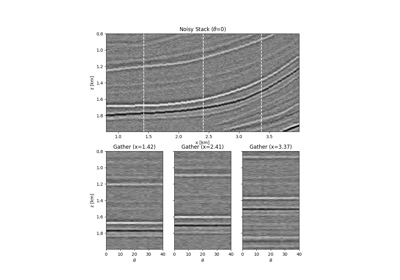# pylops.avo.prestack.PrestackInversion¶

pylops.avo.prestack.PrestackInversion(data, theta, wav, m0=None, linearization='akirich', explicit=False, simultaneous=False, epsI=None, epsR=None, dottest=False, returnres=False, epsRL1=None, **kwargs_solver)[source]

Pre-stack linearized seismic inversion.

Invert pre-stack seismic operator to retrieve a set of elastic property profiles from band-limited seismic pre-stack data (i.e., angle gathers). Depending on the choice of input parameters, inversion can be trace-by-trace with explicit operator or global with either explicit or linear operator.

Parameters: data : np.ndarray Band-limited seismic post-stack data of size $$[(n_{lins} \times) n_{t0} \times n_{\theta} (\times n_x \times n_y)]$$ theta : np.ndarray Incident angles in degrees wav : np.ndarray Wavelet in time domain (must had odd number of elements and centered to zero) m0 : np.ndarray, optional Background model of size $$[n_{t0} \times n_{m} (\times n_x \times n_y)]$$ linearization : str or list, optional choice of linearization, akirich: Aki-Richards, fatti: Fatti PS: PS or a combination of them (required only when m0 is None). explicit : bool, optional Create a chained linear operator (False, preferred for large data) or a MatrixMult linear operator with dense matrix (True, preferred for small data) simultaneous : bool, optional Simultaneously invert entire data (True) or invert trace-by-trace (False) when using explicit operator (note that the entire data is always inverted when working with linear operator) epsI : float or list, optional Damping factor(s) for Tikhonov regularization term. If a list of $$n_{m}$$ elements is provided, the regularization term will have different strenght for each elastic property epsR : float, optional Damping factor for additional Laplacian regularization term dottest : bool, optional Apply dot-test returnres : bool, optional Return residuals epsRL1 : float, optional Damping factor for additional blockiness regularization term **kwargs_solver Arbitrary keyword arguments for scipy.linalg.lstsq solver (if explicit=True and epsR=None) or scipy.sparse.linalg.lsqr solver (if explicit=False and/or epsR is not None)) minv : np.ndarray Inverted model of size $$[n_{t0} \times n_{m} (\times n_x \times n_y)]$$ datar : np.ndarray Residual data (i.e., data - background data) of size $$[n_{t0} \times n_{\theta} (\times n_x \times n_y)]$$

Notes

The different choices of cost functions and solvers used in the seismic pre-stack inversion module follow the same convention of the seismic post-stack inversion module.

Refer to pylops.avo.poststack.PoststackInversion for more details.

## Examples using pylops.avo.prestack.PrestackInversion¶08. Pre-stack (AVO) inversion